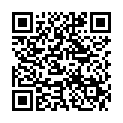## KS2 Maths Invaders - Tablet Version

Shoot the spaceship with the correct answer and dodge the incoming fire. A fun game to practise a wide range of key mathematical skills.

There are over a hundred carefully differentiated levels linked to objectives from the new maths curriculum. The game can be used to teach: Multiplication, Addition, Reading Numbers, Subtraction, Fractions of Numbers, Roman Numerals, Rounding Numbers, Division, Converting Fractions to Decimals, Converting Fractions to Percentages, Telling the Time in Words, Recognising Multiples, Factors, Prime, Square and Cube Numbers, and Simplifying Fractions. A full list of levels is below.Scan to open this game on a mobile device. Right-click to copy and paste it onto a homework sheet.### Game Objectives

You can choose to play one level, or several (from the same section).

Multiplication:

 M.1. Recall and use doubles of all numbers to 10 (Y1) M.6. 3 times table (Y3) M.11. 6 times table (Y4) M.16. Double 3 digit numbers (Y4) M.2. 2 times table (Y2) M.7. 4 times table (Y3) M.12. 7 times table (Y4) M.17. Double decimals (1dp) (Y4) M.3. 5 times table (Y2) M.8. 8 times table (Y3) M.13. 9 times table (Y4) M.18. Recognise square numbers (up to 15²) (Y5) M.4. 10 times table (Y2) M.9. Double numbers up to 100 (Y3) M.14. 11 times table (Y4) M.19. Recognise cube numbers (up to 10³) (Y5) M.5. Derive and use doubles of simple two-digit numbers (Y2) M.10. Doubles of multiples of 50 up to 500 (Y3) M.15. 12 times table (Y4) M.20. Double decimals (2dp) (Y5)

 RN.1 Read Numbers to 20 in Numerals and Words (Y1) RN.2 Read Numbers to 100 in Numerals and Words (Y2) RN.3 Read Numbers to 1000 in Numerals and Words (Y3) RN.4 Read Numbers to 10,000 in Numerals and Words (Y4) RN.5 Read Numbers to 1,000,000 in Numerals and Words (Y5) RN.6 Read Numbers to 10,000,000 in Numerals and Words (Y6)

Subtraction:

 S.1. Subtract numbers from up to 10 (Y1) S.6. Subtract from 100 (multiples of 10) (Y3) S.11. Subtract tens from a three-digit number (Y3) S.16. Subtract combinations of decimals (1dp) (Y4) S.2. Subtract numbers from up to 20 (Y1) S.7. Subtract from 100 (multiples of 5) (Y3) S.12. Subtract hundreds from a three-digit number (Y3) S.17. Subtract a decimal (2dp) from 1  (Y5 & Y6) S.3. Subtract ones from a two-digit number (Y2) S.8. Subtract from 100 (Y3 & Y4) S.13. Subtract a decimal (1dp) from 1 (Y4 & Y5) S.18. Subtract combinations of three-digit numbers (Y5 & Y6) S.4. Subtract tens from a two-digit number (Y2) S.9. Subtract from 1000 (multiples of 100) (Y3 & Y4) S.14. Subtract a decimal (1dp) from 10 (Y4 & Y5) S.19. Subtract combinations of decimals (2dp) (Y5 & Y6) S.5. Subtract a two-digit number from a two-digit number (Y2) S.10. Subtract ones from a three-digit number(Y3) S.15. Subtract two-digit numbers from three-digit numbers (y4)

Fractions of Numbers:

 FN.1. 1/2 of numbers up to 20 (whole answers) FN.5. unit fractions of up to 100 (whole answers) FN.9. harder multi-part fraction of up to 100 (e.g. 4/7 of 49) FN.2. 1/2 of numbers up to 40 (whole answers) FN.6. 1/10 of multiples of 10 (e.g. 1/10 of 60) FN.10. 1/10 of numbers up to 100 (inc. decimal answers) FN.3. 1/2 of numbers up to 100 (whole answers) FN.7. multiples of 1/10 of multiples of 10 (e.g. 3/10 of 80) FN.11. multiples of 1/10 of up to 100 (inc. decimal answers) FN.4. 1/4 of numbers up to 100 (whole answers) FN.8. simple multi-part fractions of up to 100 (e.g. 3/5 of 25) FN.12. unit fractions of up to 20 (inc. decimal answers)

Roman Numerals:

 RomN.1 Read Roman Numerals to 12 RomN.2 Read Roman Numerals to 50 RomN.3 Read Roman Numerals to 100 RomN.4 Read Roman Numerals to 1000 RomN.5 Recognise years written in Roman Numerals

Rounding Numbers:

 RoundN.1. Round numbers up to 100 to the nearest 10 (Y2) RoundN.2. Round numbers up to 1000 to the nearest 10 (Y3) RoundN.3. Round numbers up to 10,000 to the nearest 10, 100 or 1000 (Y4) RoundN.4. Round decimals with one decimal place to the nearest whole number (Y4) RoundN.5. Round decimals with two decimal places to the nearest whole number and to one decimal place (Y5)

Division:

 D.1. Half of numbers to 20 (Y1) D.5. Derive and use halves of simple two-digit even numbers (Y2) D.9. Halve numbers up to 200 (Y3) D.13. Division facts for the 11 times table (Y4) D.2. Division facts for the 2 times table (Y2) D.6. Division facts for the 3 times table (Y3) D.10. Division facts for the 6 times table (Y4) D.14. Division facts for the 12 times table (Y4) D.3. Division facts for the 5 times table (Y2) D.7. Division facts for the 4 times table (Y3) D.11. Division facts for the 7 times table (Y4) D.15. Halve decimals (1dp) (Y4) D.4. Division facts for the 10 times table (Y2) D.8. Division facts for the 8 times table (Y3) D.12. Division facts for the 9 times table (Y4) D.16. Halve decimals (2dp) (Y5)

Convert Fractions to Decimals:

 CFP.1. Unit Fractions (e.g. 1/2, 1/4, 1/5, 1/10, 1/100) CFP.2. Multi-part Fractions (e.g. 3/4, 2/5, 7/10, 58/100) CFP.3. Harder Unit Fractions (as per CFP.1. plus 1/3, 1/6, 1/8) CFP.4. Harder Multi-part Fractions (e.g. 2/3, 5/6, 3/8) CFP.5. Improper Fractions

Convert Fractions to Percentages:

 CFD.1. Unit Fractions (e.g. 1/2, 1/4, 1/5, 1/10, 1/100) CFD.2. Multi-part Fractions (e.g. 3/4, 2/5, 7/10, 58/100) CFD.3. Harder Unit Fractions (as per CFD.1. plus 1/3, 1/6, 1/8) CFD.4. Harder Multi-part Fractions (e.g. 2/3, 5/6, 3/8) CFD.5. Improper Fractions

Telling the Time in Words:

 TTW.1. Read time to the hour and half hour TTW.2. Read time to a quarter hour TTW.3. Read time to the nearest 5 mnutes TTW.4. Read time to the minute

Multiples, Factors, Prime, Square and Cube Numbers:

 MFPSC.1. Multiples of up to 12 MFPSC.2. Factors of numbers up to 40 MFPSC.3. Prime numbers MFPSC.4. Square numbers